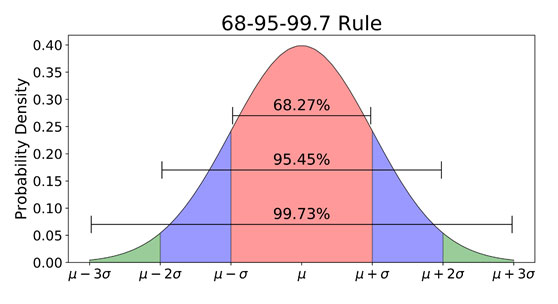# Outliers and How to Deal With Them

posted in: Blog | 0

Introduction

Continuous societal development has brought about numerous disruptive phenomena to society that have redefined the organization of virtually every sphere of life. One such disruption is in the field of math and statistics-data revolution. There is a phrase that goes, “data is the new oil” which essentially implies that organizations are starting to recognize the value of data in defining their value proposition. However, leveraging the power of data in decision-making is not as simple as it sounds owing to the large volumes of data being generated every single day. As such, this concern begs the question, “How then does one make sense of all this data?”

Statistics –the science of collecting, analyzing, presenting, and interpreting data- has a lot of cumbersome mathematical underpinnings that inform its use in analytical processes. However, its application is supposed to communicate a story about some particular dataset in terms of the defined measures.  In this brief discussion, we look at some of the simplest analytical measures that are used in data analysis.

Mean/average

One of the most intriguing things about the data collected from real-world events is its unevenness. For example, take data collected on students, it’s almost impossible to find identical students on all the data points that you collect. However, if you were to observe the data collected, for instance, narrow it down to one of the data points say age, you find a lot of similarities. Assuming that the chosen sample is a university class, it is possible to find that the majority of students fall between 18 and 24 years of age. Additionally, most students’ age will likely lie towards the middle of this range, say 21. This number (21) is referred to as the mean/average age or a central point in the data and forms one of the most important measures that most analysts use. Interestingly, in different societal spheres like the media, political scene, and academia, the mean or average measure of a particular data is commonly used to paint an image of how such data looks.

Standard deviation

Another important measure is how the data varies from the mean. Suppose one is interested in determining the manner in which specific data points vary/deviate from the determined average, we calculate the standard deviation. These two metrics simply show how dispersed the data is in relation to the mean. In fact, statisticians say that you only need these two measures (mean and standard deviation) to describe any data that is regular or is in a standard format. For instance, it is possible to deduce that 67% of the data lie one unit away from the mean. For instance, suppose we pick a random sample of college students whose average age is 21 years, we can rightly infer that about  67% of them are between 20 and 22 years old if we know the standard deviation is 1.

Normality in data

It is also possible to conclude that 95% of the students have ages between two standard deviations from the mean which is between 19 years and 22 years. This relationship is represented by what we call a normal distribution curve or the bell curve.Outliers